# Is it true that every recursively enumerable language is recursive, and is it true that every...

Is it true that every recursively enumerable language is recursive, and is it true that every language is recursively enumerable?

When the language is recursive, there will exist the Turing machine which can accept or reject the language. The recursive languages will be the subset of recursively enumerable, thus the Turing recognizable means that the language is also recursively enumerable. Thus it is true that the recursively enumerable language or the recursive languages are always recursively enumerable.

**Please Hit Like if you appreciate my answer. For further doubts on the or answer please drop a comment, I'll be happy to help. Thanks for posting.**

#### Earn Coin

Coins can be redeemed for fabulous gifts.

Similar Homework Help Questions
• ### Question 3 3 pts There are NDTMs for which there is no equivalent DTM. True O...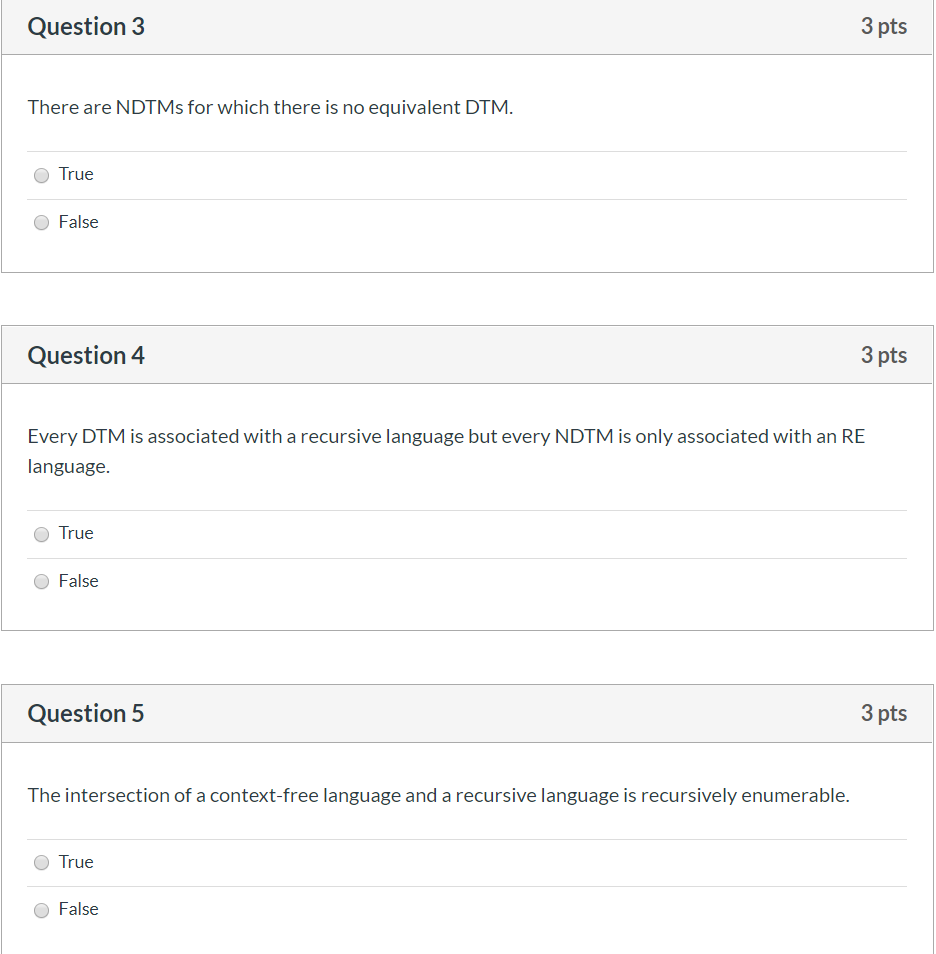Question 3 3 pts There are NDTMs for which there is no equivalent DTM. True O False Question 4 3 pts Every DTM is associated with a recursive language but every NDTM is only associated with an RE language. O True O False Question 5 3 pts The intersection of a context-free language and a recursive language is recursively enumerable. O True False

• ### Suppose the language L ? {a, b}? is defined recursively as follows: ? L;    for every...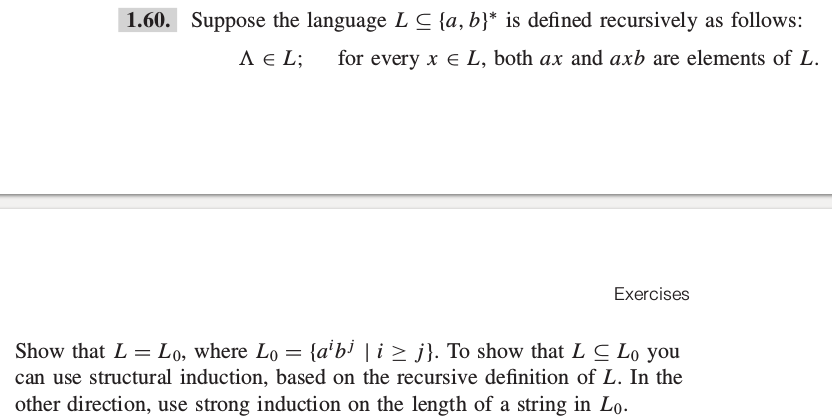Suppose the language L ? {a, b}? is defined recursively as follows: ? L;    for every x ? L, both ax and axb are elements of L. Show that L = L0 , where L0 = {aibj | i ? j }. To show that L ? L 0 you can use structural induction, based on the recursive definition of L. In the other direction, use strong induction on the length of a string in L0. 1.60. Suppose the language...

• ### Determining whether languages are finite, regular, context free, or recursive 1. (Each part is worth 2 points) Fill in the blanks with one of the following (some choices might not be used): a) finit...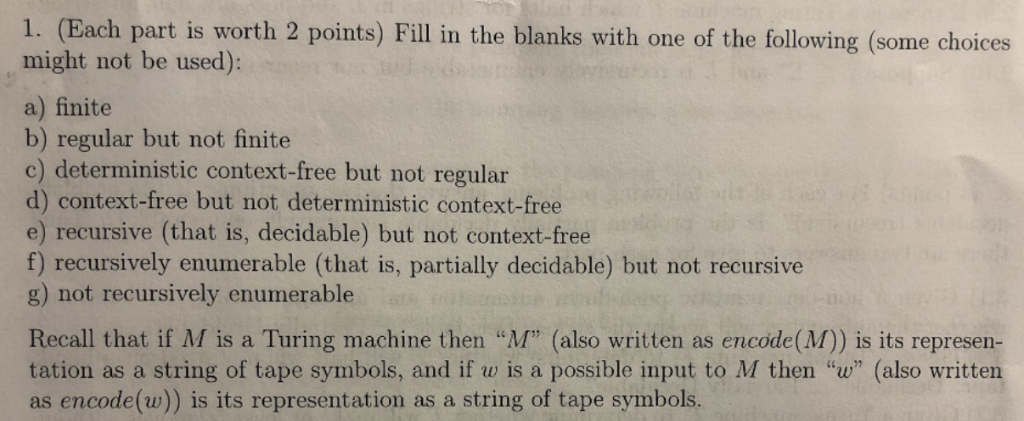Determining whether languages are finite, regular, context free, or recursive 1. (Each part is worth 2 points) Fill in the blanks with one of the following (some choices might not be used): a) finite b) regular but not finite d) context-free but not deterministic context-free e) recursive (that is, decidable) but not context-free f) recursively enumerable (that is, partially decidable) but not recursive g) not recursively enumerable Recall that if M is a Turing machine then "M" (also written as...

• ### Question 9. Consider the language {a"b" : n >0}. (i) Is this a regular language? Why...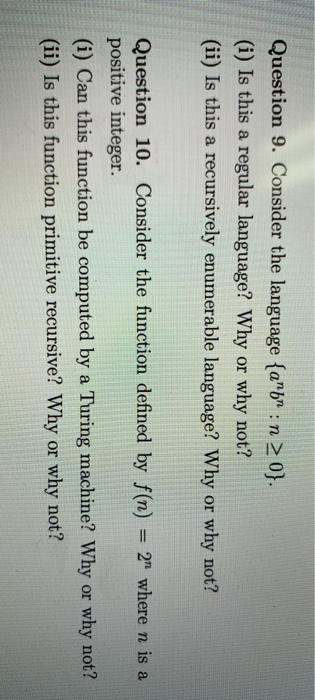Question 9. Consider the language {a"b" : n >0}. (i) Is this a regular language? Why or why not? (ii) Is this a recursively enumerable language? Why or why not? Question 10. Consider the function defined by f(n) = 2 where n is a positive integer. (i) Can this function be computed by a Turing machine? Why or why not? (ii) Is this function primitive recursive? Why or why not?

• ### Computer Theory 3. (a) Prove that the language LH IR(M) w machine M halts with input...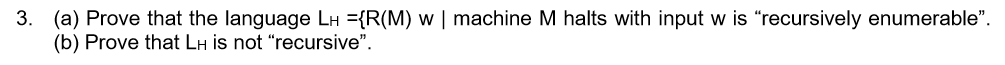Computer Theory 3. (a) Prove that the language LH IR(M) w machine M halts with input w is "recursively enumerable" (b) Prove that LH is not "recursive"

• ### please solve the problems(True/False questions) 25. There is a problem solvable by Turing machines with two...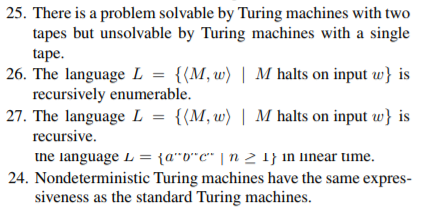please solve the problems(True/False questions) 25. There is a problem solvable by Turing machines with two tapes but unsolvable by Turing machines with a single tape 26. The language L = {(M, w) | M halts on input w} is recursively enumerable 27. The language L = {(M,w) | M halts on input w is recursive ne language L = {a"o"c" | n 2 1} in linear time 24. Nondeterministic Turing machines have the same expres siveness as the standard...

• ### Consider the language { a nb n: n ≥ 0 } . (i) Is this a...

Consider the language { a nb n: n ≥ 0 } . (i) Is this a regular language? Why or why not? (ii) Is this a recursively enumerable language? Why or why not?

• ### Question 9. Consider the language {a^n b^n : n ≥ 0}. (i) Is this a regular...

Question 9. Consider the language {a^n b^n : n ≥ 0}. (i) Is this a regular language? Why or why not? (ii) Is this a recursively enumerable language? Why or why not?

• ### If L is recursive, is it necessarily true that L^+ is also recursive? Peter Lin: Formal...

If L is recursive, is it necessarily true that L^+ is also recursive? Peter Lin: Formal Language and Automata